Lydia’s idea emerged when I was teaching multiplication in her third grade class. To engage the students with connecting real-world situations to multiplication, and also with estimating and calculating accurate answers in several different ways, I presented a word problem: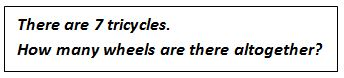The students wrote a multiplication equation, estimated, and then figured out the answer. We discussed their different strategies for computing and agreed that 7 x 3 = 21.

I then gave a related assignment. This time I presented the students with a multiplication equation―8 x 4 = ? I asked them to estimate the product, write a word problem that matched the equation, and figure out the answer in at least two different ways.

As I circulated, Lydia was eager to explain to me how she had arrived at the estimate of 32. She had noticed that one less than each of the factors in 8 x 4 resulted in 7 x 3, the factors in the tricycle problem we had solved. So she figured that the answer to 8 x 4 should follow the same pattern, and she changed 21 to 32.  Here’s what she wrote.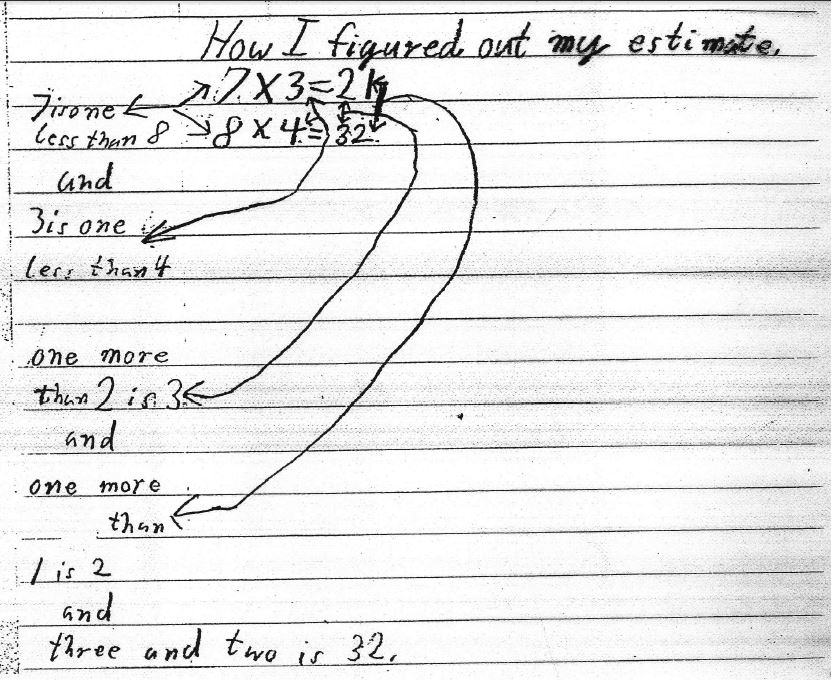I was astonished. And speechless. (I think it’s hard to teach and think at the same time, especially when we experience unexpected responses from students.)

Lydia happily continued with the assignment. She wrote an appropriate word problem―There were 8 dancers. Each dancer held 4 canes. How many canes all together? When she solved the problem (she did this in five different ways), she was pleased that her estimate was the exact answer.

Meanwhile, I was wondering about what Lydia had done and why it had worked. I grabbed a piece of paper and tried her method on other problems, increasing each factor and the digits in the product by 1 . It didn’t work the first few times, but then it worked again!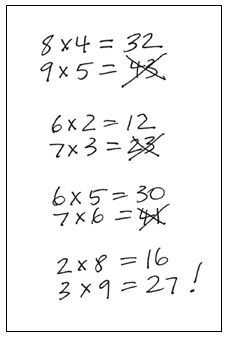I returned to Lydia and asked her to try her method on 6 x 2, a problem I knew wasn’t too hard for her to figure. Also, the factors were each 1 less than in 7 x 3, so it related back to the tricycle problem. Lydia wrote 6 x 2 = 12 and, underneath, increased each digit by 1, producing the incorrect result that 7 x 3 = 23.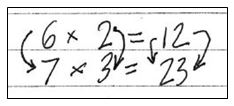“Hey,” she said, “it wouldn’t work. I don’t get it. It worked over here.” She referred again to how she had estimated 8 x 4 from 7 x 3.

While I found Lydia’s conjecture surprising and intriguing, I discounted it as just a lucky occurrence . . . until I investigated further.

That night, I phoned a math friend (Nicholas Branca, who was a mathematical marvel) and told him what had happened. I gave him my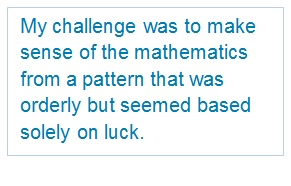conclusion that Lydia’s reasoning had no mathematical grounding, even though it worked at times. Nicholas’s response was, “If a student does something in an orderly way, following a clear and predictable pattern, then it’s likely that there’s some mathematical grounding. I’ll get back to you.” (Let’s hear it for the Common Core Mathematical Practice Standard 7: Look for and make use of structure.)

I spent a good deal of time that night trying Lydia’s method on problems with different pairs of factors, looking for patterns relating to when her method did and didn’t work, and trying to figure out why. Nicholas and I talked several times.

I love investigations like these, where the math isn’t particularly difficult or complex so the problem is accessible, but there’s something mathematically wonderful, and often surprising and delightful, to discover.

I’m now convinced that Lydia’s reasoning indeed has mathematical grounding, that it’s possible to predict when her method will and won’t work, and to be able to explain why. Interested in finding out more? Try these investigations.

Suggestions for Investigating
The investigations below helped me make sense of the mathematics underlying Lydia’s method. I’ve used the first two suggestions with third, fourth, and fifth graders.

More recently, I put together the full list of investigations for a professional learning session. Teachers were free to investigate some or all of them, and in any order. Then, aftereveryone had some time to think by themselves, I organized them into areas in the room numbered for the seven suggestions. They had the choice to continue working on their own or to join a group that was focusing on the same question. It was OK for them to switch to another group as they worked. I think that having choices about what to investigate and how is valuable, both for professional learning sessions and in classrooms with students. I think this would be a good set of investigations and structure for a middle school or high school class.

1. Does Lydia’s method work for other pairs of equations? Why?
2. What can you discover about pairs of equations for which it does and doesn’t work?
3. How might using rectangular arrays inform the problem?
5. How can you use algebra to explain what’s happening?
6. What happens with negative factors?
7. What about factors that aren’t whole numbers; e.g., 2.6 or 3.05?

As always, I’m interested in any feedback and/or questions.

•Joshua says:

Another exploration, if you want to add to the list: working in other bases.
For example, “Lydia’s method” does work for 6×2, just do the calculations in octal. In base 8:
6×2 = 14 (base 8)
7×3 = 25 (base 8)

But then it doesn’t work when we take the next step to 8×4 (or 10 x 4 in our base 8 representation) and it also doesn’t work if we go backwards to 5×1.

These are fun investigations, but I would be reluctant to call this a method because that makes it sound like a “method for multiplication” and the conditional “if m x n = 10a+b, then (m+1) x (n+1) = 10 (a+1) + (b+1)” is not true (it becomes true if additional conditions are placed on m and n, as per your suggested investigations).

Joshua
3jlearneng.blogspot.com

•Marilyn Burns says:

Maybe I should characterize it as a conjecture, not as a method? Thanks for your suggestion for another exploration. I’ll add it to my list.

•Judith says:

I love your work, but often feel left behind the curve in figuring out how to use some of the examples with my students. Take this May 11th post, in example: Have you found proof to support this method as a sound form for the student to use? I don’t see a consistency in pattern that would be viable for this student. What am I not understanding?

•Marilyn Burns says:

See Joshua’s comment and my reply. It’s not a reliable method in that it doesn’t always work. But figuring out when it does work is a mathematical investigation that I think is valuable for students. I ask students, “Search for problems where it does work and where it doesn’t, and look for patterns.” (There is a consistent pattern.) I start a two-column class chart for problems that “work” (7 x 3 to 8 x 4, e.g.) and problems that “don’t work” (6 x 2 to 7 x 3) and students record examples. Questions: Can you predict before trying whether it will work? Why do some work and others don’t? Does this help?

•Chaya says:

Using an array was particularly helpful, as it helped me realize that this “rule” will work only when the array increases by 11 squares. For example, changing from 7×3 to 8×4 adds 11 squares to the array – 7 along one side, 3 along the other, and 1 in the corner. Lydia’s rule works for other multiplication problems in which the factors in the first problem have a sum of 10: 2×8, 4×6, 5×5…but not 9×1.

This is a wonderfully rich problem that could work at many grade levels, as it could be modeled in many ways. It also gives credit to a student’s discovery, while really digging into why it works and asking that most important mathematical question: will it work every time?

•Chaya says:

Actually, 9×1 does work. However, the increase in the tens place and ones place probably wouldn’t be obvious to a 3rd grader (21 –> 32 is obvious, while 9 –> 20 may not be).

•Jennifer says:

Interesting that the relationship between the two factors that result in the initial product is that they must have a sum of 10 (going to explore further, but here is reasoning so far):

Given: ab = 10t + s

If (a + 1)(b + 1) = 10(t + 1) + (s + 1) is true, then
ab + a + b + 1 = (10t + s) + 11
ab + a + b + 1 = ab + 11
a + b + 1 = 11
a + b = 10

•Samriddha says:

I was about to comment on the same observation (sum of 10).
Also, combined with Joshua’s observation above, here’s my conclusion:

This method applies for base n if sum of numbers in first products is n.
Which also brings us to the conclusion that it won’t apply for more than two consecutive sets for the same base.

•Marsha W. Lilly says:

What a rich investigation to explore for students! (and teachers)
I plan to use this problem with my Middle School Math Club students tomorrow…
Judith – Why do you feel the need to justify a rich problem?
More can be gained from providing a conjecture than by solving problems we know that “work.”

•Tyler says:

Looks like a number of folks beat me to the solution. Figuring out when it works is relatively simple if you are comfortable setting it up and solving it algebraically (as Jennifer showed), but I really like your suggestion of drawing an array.

One aspect of teaching that I really enjoy is trying to find solutions that are more accessible for students. Using Algebra is a straightforward process here, but it is not essential to investigating this pattern. In fact, I think this is a richer investigation when students aren’t comfortable using Algebra. Using an array really helps show why the factors must have a sum of 10.

I also did some exploring with negative factors and was surprised by what happened! At first I couldn’t figure out why I got what I did…but then…!!!

I really like investigations like this when students are studying properties (ex. properties of exponents). It is fun to present them with a list of “potential properties” and have them figure out which ones are always true, almost always true, almost always false, and false. This helps them see why we describe some relationships as properties but others are left off the list. For example, a^b=b^a isn’t listed as a property of exponents, but is it sometimes true? This experience also makes those relationships feel less abstract.

•Carol Treglio says:

Very cool, it works only when the original digits being multiplied have a sum of 10.

•Dan says:

Very cool question–I’m kind of astonished I’ve never heard a student pose it before!

Not sure if the higher digit version is quite as compelling, because not all the digits on the left side get larger, but…
7 x 103 = 721
8 x 104 = 832

•Dan says:

On second thought, that’s kind of a trivial addition to the problem.

23 x 987 = 22,701
24 x 988 = 23,712

It seems like we can’t make all the digits go up with larger numbers.

•MQ says:

This is an arithmetic way of exploring what Chaya describes in terms of arrays.

Consider increasing each of the digits 7 and 3 in the product 7 x 3.

When it becomes 8 x 3, we have one more 3: so we have added 3.

When it then becomes 8 x 4, we have one more 8: so we have added 8 to our 3.

In total, we have added 3+8 = 11.

Instead, we might’ve changed our digits in the other order:

7 x 4 means we have one more 7: so we have added 7.

When it becomes 8 x 4, we have one more 4: so we have added 4 to our 7.

In total, we have added 7+4 = 11.

Since adding 11 increases each digit in a two-digit number, our product changes as desired.

Given this insight about 11, let’s try working backwards.

How might we have found 5+6, another expression for 11, being added on?

Adding 5 could correspond to increasing our 5 count by one:

5 x D becomes 5 x (D+1).

Next, we change this to (5+1) x (D+1).

Since the latter change corresponds to increasing our total by 6, we have D+1 = 6.

So D = 5, and the recovered insight is:

5×5 = 25. Increasing each digit by 1,
6×6 = 36.

The method described above can be used to investigate others’ observations about the digits adding up to 10.

•Mochi says:

I wrote down some multiplication problems;

8×3 =24
+12 (example 3+9 or 8 +4)
9×4=36
+14 (4+10 or 9 + 5)
10×5=50

Then I used area model of each problem. Then I found out that the answer need to add one of the factor from previous and current to get current product. That’s why Lidia’s method only worked when the sum of the factor become 11. Which I can use this patter to find our all the combination of product.
1 X9= 9
2X10=20

2X8 = 16
3X9=27

3×7=21, 4X6=24, 5×5 =25 , 6×4 =24, 7×3=21, 8×2=16, 9×2=18, 9×1=9, 10×0 =0

I only did up to question 3 at this time (I’s getting ready to go to work). I am teaching 4th and 5th (loop) grade and try to think like 9-11 years old not adults to make sense so that I can facilitate their learning.

I took math solution workshops about four weeks total. I loved every single moment even though I agitated facilitators by asking questions or sharing various ways to solve each problem:-) I wish all children have environment to explore mathematical idea connecting their prior knowledge to make sense in their own way instead of practicing the same skills repeatedly without questioning reasons behind what they are doing.

•Marilyn Burns says:

I did something similar, using rectangular arrays for an area model. But I looked at it a little differently. For changing 8 x 3 to 9 x 4, I increased the product of 8 x 3 by 8 + 3 + 1, with the 1 being the corner of the array. I’m not sure my drawing below helps explain how I was thinking, so let me know. But I used the same “add the two factors and then one more for the corner” for the others, too. For 1 x 9 to 2 x 10, I added 1 + 9 + 1 to the initial product of 9 to get 20. For 2 x 8 to 3 x 9, I added 2 + 8 + 1 to the initial product of 16 to get 27. Thanks, also, for the feedback on the Math Solutions courses.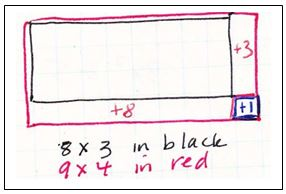•Richard Pond says:

Here’s a crazy concept. Why don’t we make sure that all students memorize single digits 2-9, in addition, subtraction, multiplication and division. This way they can use it in the real world for everything they do. Thanks!

•Marilyn Burns says:

I don’t have a quarrel about students knowing the basic single digit sums and products. I just want to be sure that a focus on memorizing doesn’t precede or supplant a focus on understanding.